Ex 7.3

Chapter 7 Class 11 Permutations and Combinations
Serial order wise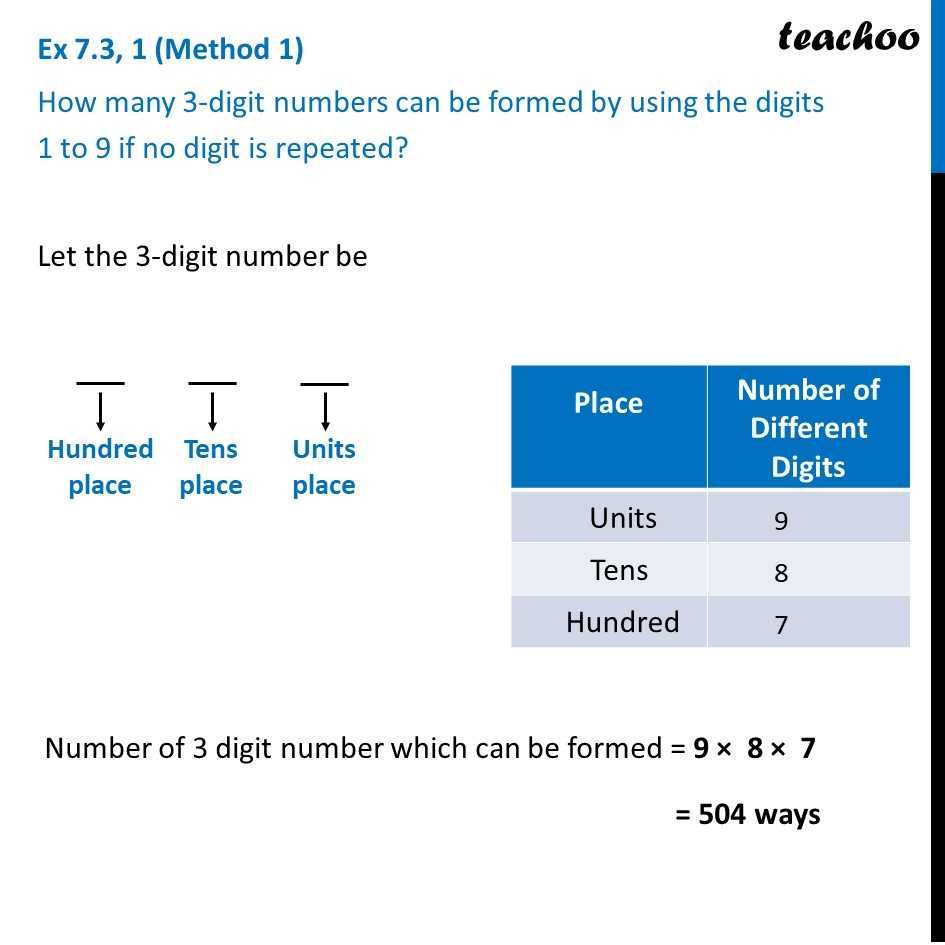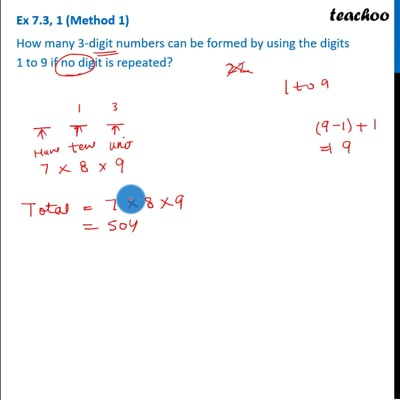This video is only available for Teachoo black users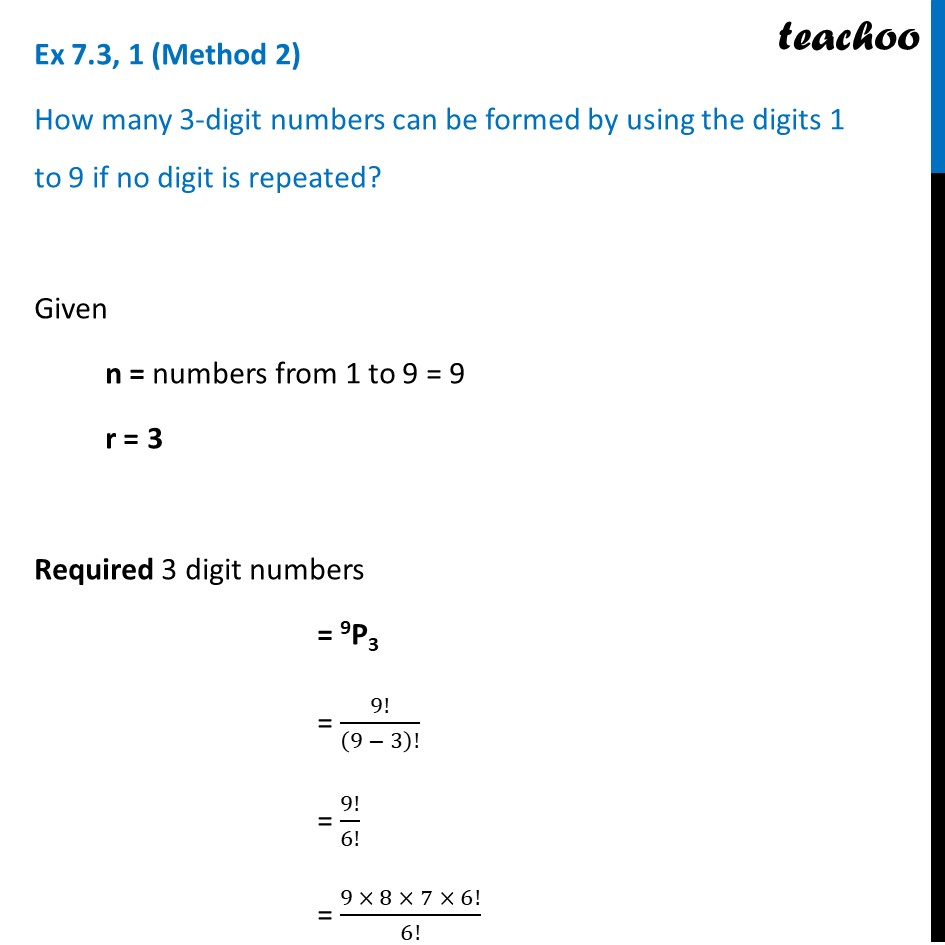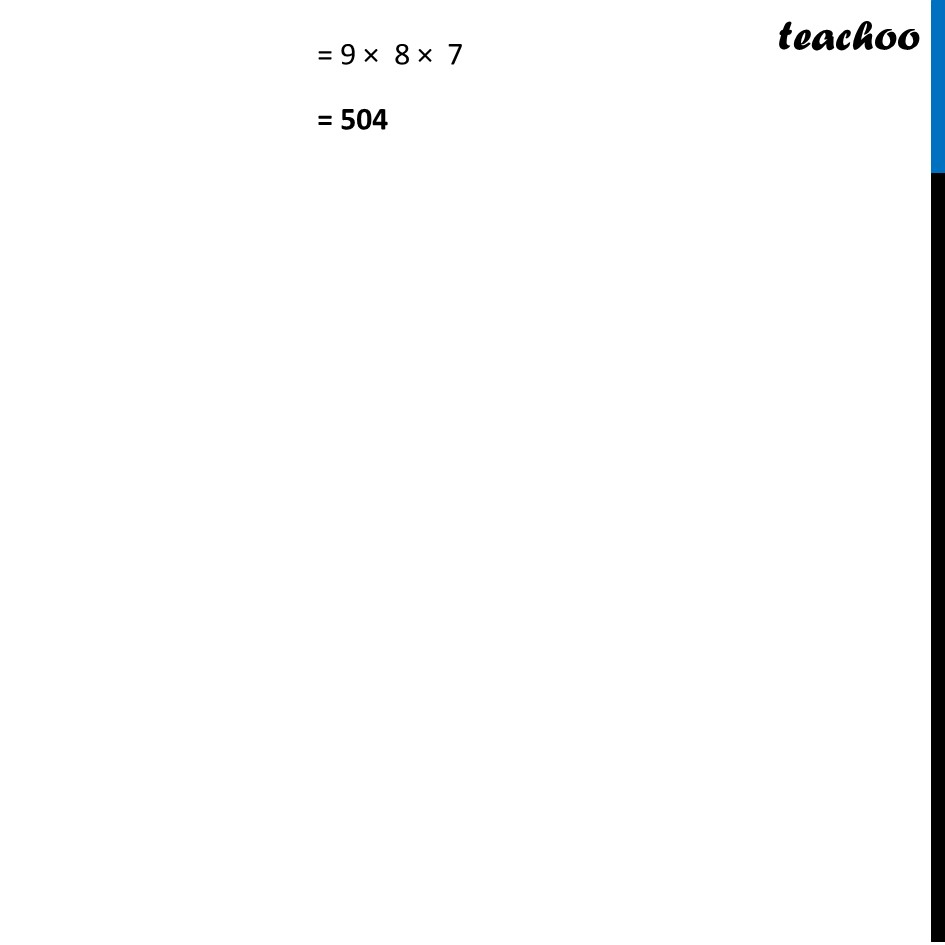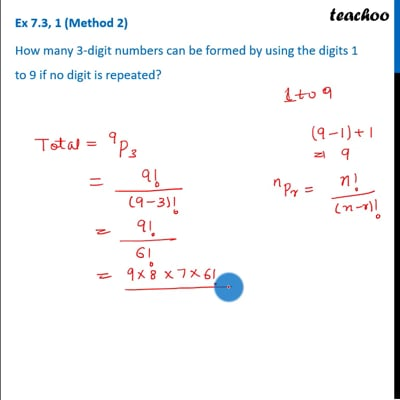This video is only available for Teachoo black users

Introducing your new favourite teacher - Teachoo Black, at only ₹83 per month

### Transcript

Ex 7.3, 1 (Method 1) How many 3-digit numbers can be formed by using the digits 1 to 9 if no digit is repeated? Let the 3-digit number be Number of 3 digit number which can be formed = 9 × 8 × 7 = 504 ways Ex 7.3, 1 (Method 2) How many 3-digit numbers can be formed by using the digits 1 to 9 if no digit is repeated? Given n = numbers from 1 to 9 = 9 r = 3 Required 3 digit numbers = 9P3 = 9!/(9 − 3)! = 9!/6! = (9 × 8 × 7 × 6!)/6! = 9 × 8 × 7 = 504